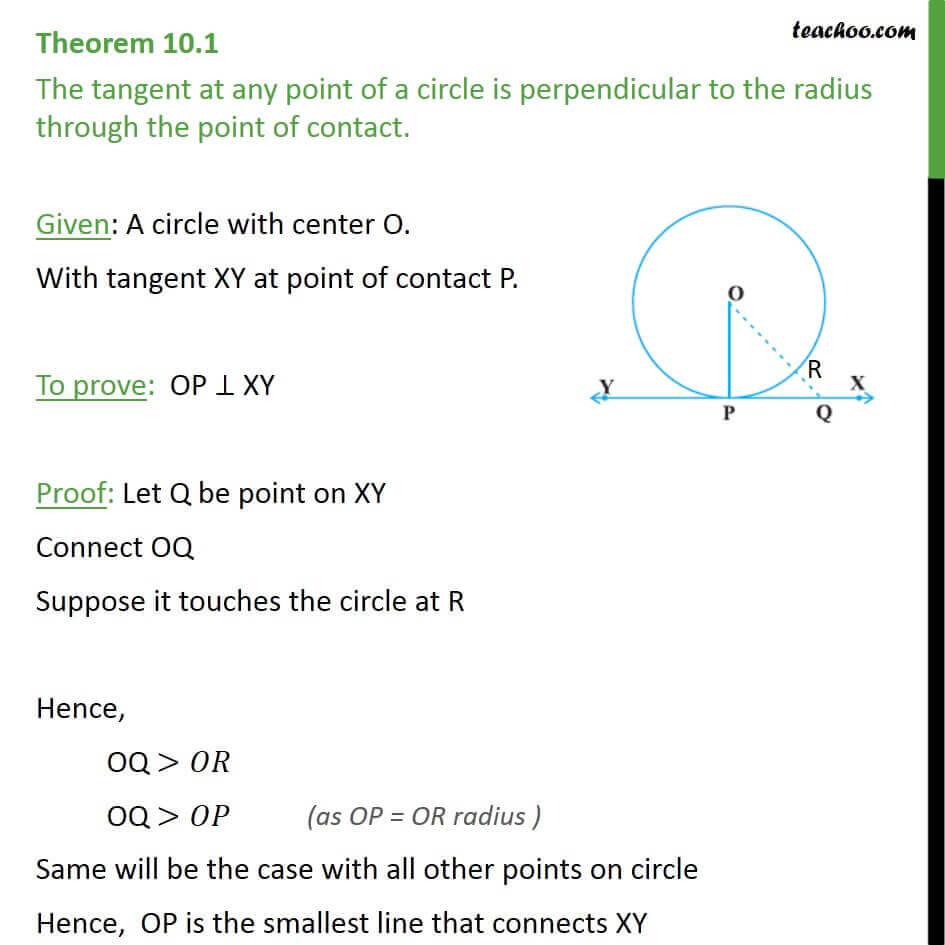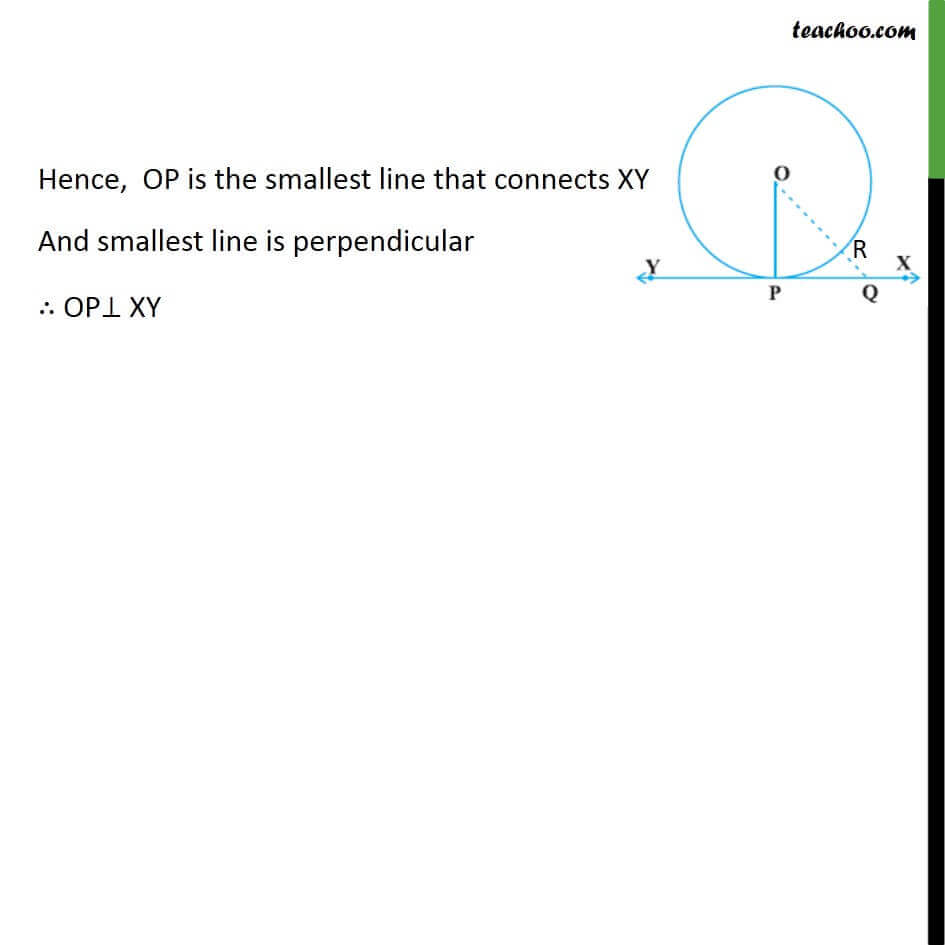Therorems

Chapter 10 Class 10 Circles
Serial order wiseLearn in your speed, with individual attention - Teachoo Maths 1-on-1 Class

### Transcript

Theorem 10.1 The tangent at any point of a circle is perpendicular to the radius through the point of contact. Given: A circle with center O. With tangent XY at point of contact P. To prove: OP ⊥ XY Proof: Let Q be point on XY Connect OQ Suppose it touches the circle at R Hence, OQ >𝑂𝑅 OQ >𝑂𝑃 Same will be the case with all other points on circle Hence, OP is the smallest line that connects XY Hence, OP is the smallest line that connects XY And smallest line is perpendicular ∴ OP⊥ XY